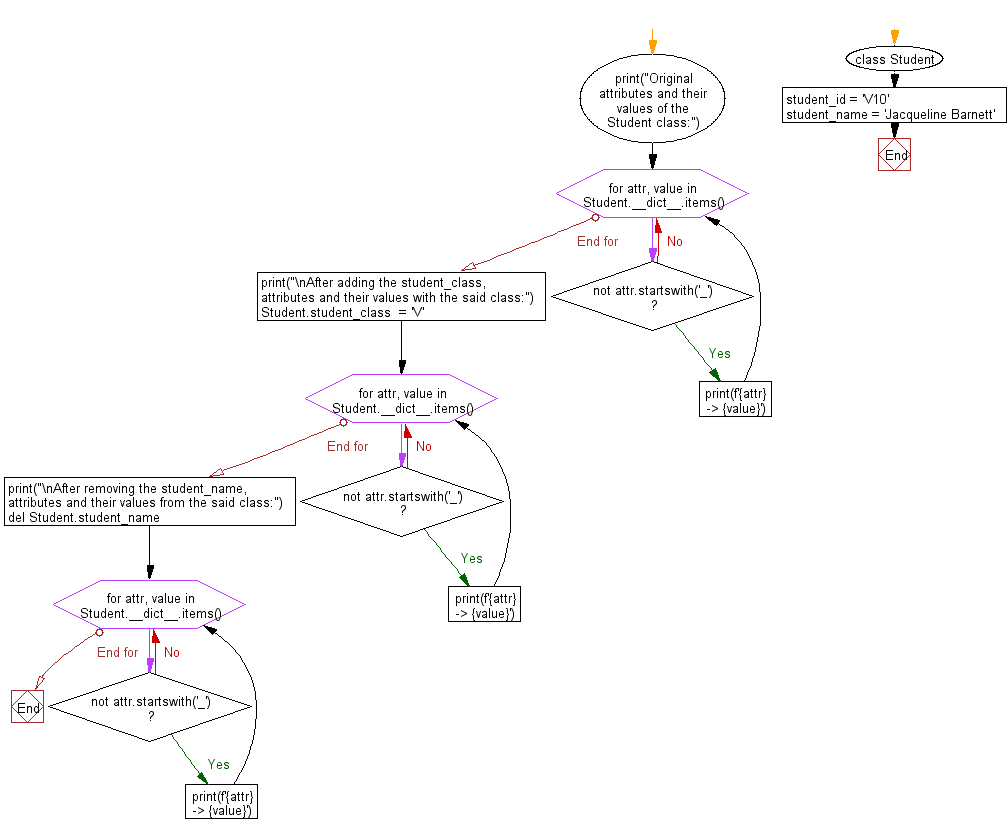﻿ Python: Add and remove attribute in a given class - w3resource# Python: Add and remove attribute in a given class

## Python Class ( Basic ): Exercise-10 with Solution

Write a Python class named Student with two attributes student_id, student_name. Add a new attribute student_class and display the entire attribute and their values of the said class. Now remove the student_name attribute and display the entire attribute with values.

Sample Solution:

Python Code:

``````class Student:
student_id = 'V10'
student_name = 'Jacqueline Barnett'
print("Original attributes and their values of the Student class:")
for attr, value in Student.__dict__.items():
if not attr.startswith('_'):
print(f'{attr} -> {value}')
print("\nAfter adding the student_class, attributes and their values with the said class:")
Student.student_class  = 'V'
for attr, value in Student.__dict__.items():
if not attr.startswith('_'):
print(f'{attr} -> {value}')
print("\nAfter removing the student_name, attributes and their values from the said class:")
del Student.student_name
#delattr(Student, 'student_name')
for attr, value in Student.__dict__.items():
if not attr.startswith('_'):
print(f'{attr} -> {value}')
```
```

Sample Output:

```Original attributes and their values of the Student class:
student_id -> V10
student_name -> Jacqueline Barnett

After adding the student_class, attributes and their values with the said class:
student_id -> V10
student_name -> Jacqueline Barnett
student_class -> V

After removing the student_name, attributes and their values from the said class:
student_id -> V10
student_class -> V
```

Flowchart:## Visualize Python code execution:

The following tool visualize what the computer is doing step-by-step as it executes the said program:

Python Code Editor:

What is the difficulty level of this exercise?

Test your Programming skills with w3resource's quiz.

﻿

## Python: Tips of the Day

The Zip() Function:

```>>> students = ('John', 'Mary', 'Mike')
>>> ages = (15, 17, 16)
>>> scores = (90, 88, 82, 17, 14)
>>> for student, age, score in zip(students, ages, scores):
...     print(f'{student}, age: {age}, score: {score}')
...
John, age: 15, score: 90
Mary, age: 17, score: 88
Mike, age: 16, score: 82
>>> zipped = zip(students, ages, scores)
>>> a, b, c = zip(*zipped)
>>> print(b)
(15, 17, 16)
```